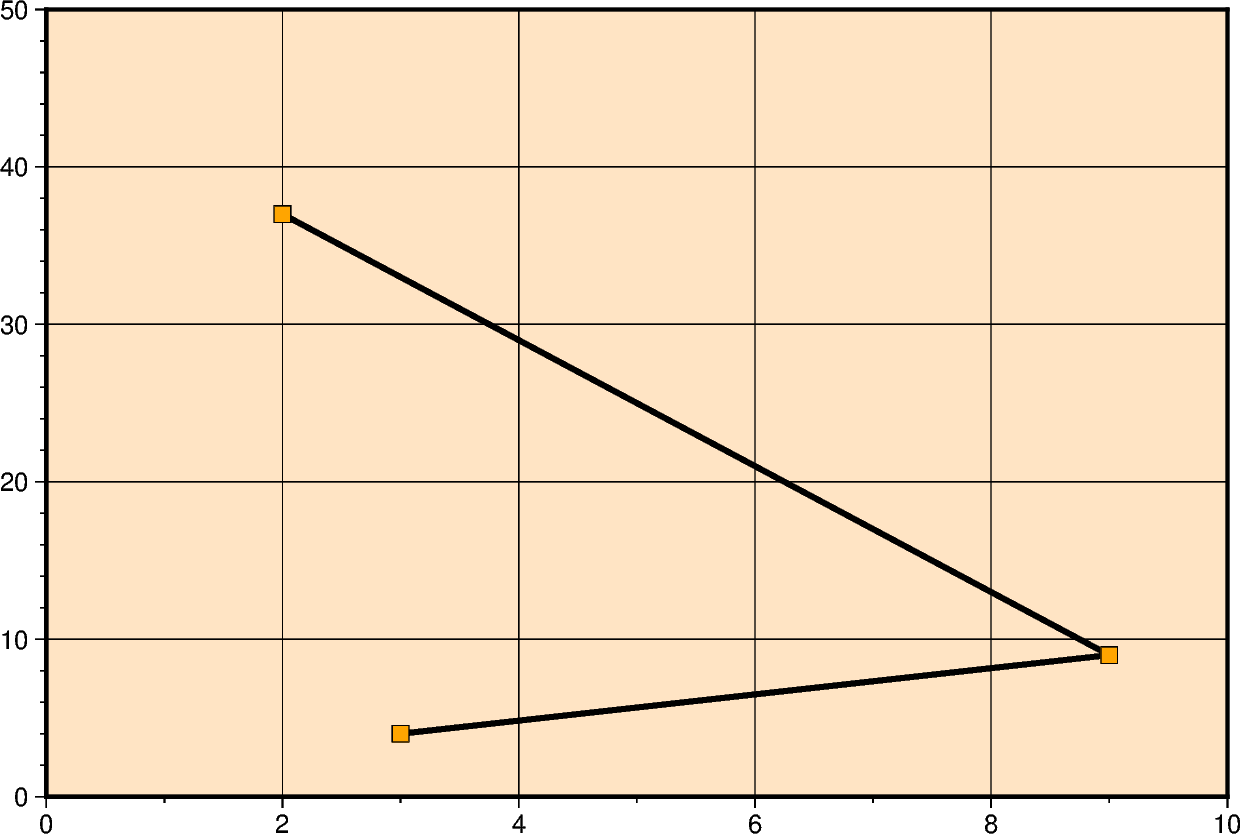# Cartesian linear

Xwidth[/height] or xx-scale[/y-scale]

Give the width of the figure and the optional height. The lower-case version x is similar to X but expects an x-scale and an optional y-scale.

The Cartesian linear projection is primarily designed for regular floating point data. To plot geographical data in a linear projection, see the upstream GMT documentation Geographic coordinates. To make the linear plot using calendar date/time as input coordinates, see the GMT documentation Calendar time coordinates.```import pygmt

fig = pygmt.Figure()
fig.plot(
# The x and y parameters determine the coordinates of lines
x=[3, 9, 2],
y=[4, 9, 37],
pen="3p,red",
# The region parameter sets the x and y ranges of the
# Cartesian projection
region=[0, 10, 0, 50],
projection="X15c/10c",
# "WSne" is passed to the frame parameter to put annotations
# only on the left and bottom axes
frame=["af", "WSne"],
)
fig.show()
```

Total running time of the script: (0 minutes 0.519 seconds)

Gallery generated by Sphinx-Gallery